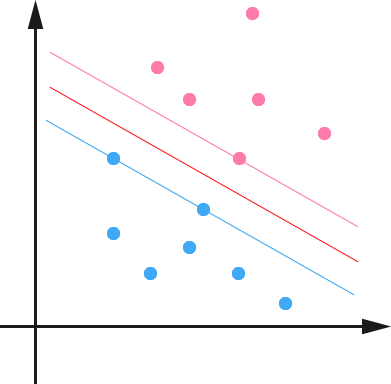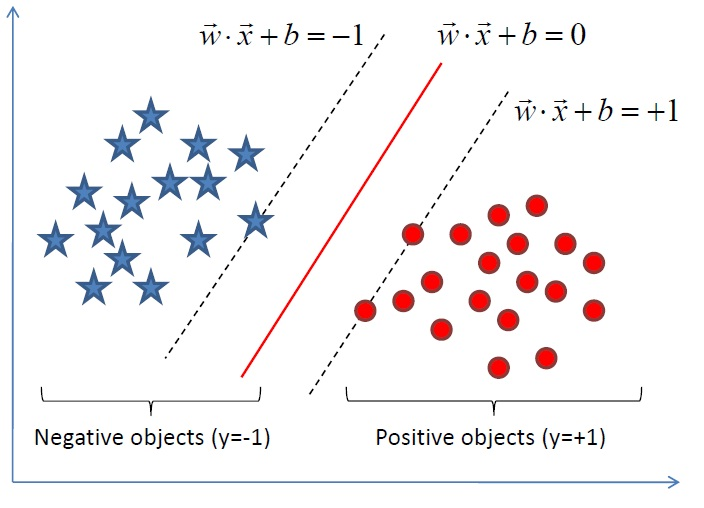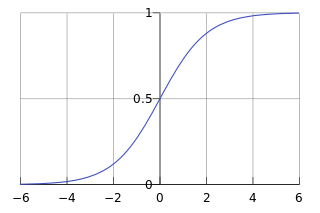# 常用分类算法

## Bayes

### 朴素贝叶斯的优缺点

• 优点
1. 所需估计的参数少，对于缺失数据不敏感。
• 缺点
1. 假设属性之间相互独立，这往往并不成立。（喜欢吃番茄、鸡蛋，却不喜欢吃番茄炒蛋）。
2. 需要知道先验概率。
3. 分类决策错误率。

### 朴素贝叶斯的公式

• 朴素贝叶斯求解：
P(C|F1,...,Fn)=p(C)p(F1,...,Fn|C)p(F1,...,Fn)=p(C)i=1np(Fi|C)

## Decision Tree

决策树代码

### 决策树的优缺点

• 优点
1. 不需要任何领域知识或参数假设。
2. 适合高维数据。
3. 简单易于理解。
4. 短时间内处理大量数据，得到可行且效果较好的结果。
• 缺点
1. 对于各类别样本数量不一致数据，信息增益偏向于那些具有更多数值的特征。
2. 易于过拟合。
3. 忽略属性之间的相关性。
4. 不支持在线学习

### 决策树公式

• 熵：
Entropy(S)=pilogpi
• 信息增益：
Entropy(S,A)=Entropy(S)vV(A)|Sv||S|Entropy(Sv)
• 分裂信息：
SplitInfoR=j=1k|Dj||D|log2|Dj||D|
• 增益比率：
GainRatio(R)=Gain(R)SplitInfoR(D)
• 基尼指数：
Gini(S)=1imp2i

## SVM

### 支持向量机的优缺点

• 优点
1. 可以解决小样本下机器学习的问题。
2. 提高泛化性能。
3. 可以解决高维、非线性问题。超高维文本分类仍受欢迎。
4. 避免神经网络结构选择和局部极小的问题。
• 缺点
1. 缺失数据敏感。
2. 内存消耗大，难以解释。
3. 运行和调差略烦人。

### 支持向量机的公式max1||ω||s.t.,yi(ωT+b)1,i=1,...,n

max1||ω||2s.t.,yi(ωT+b)1,i=1,...,n

Lω=0ω=i=1nαiyixi

Lb=0i=1nαiyi=0

L(ω,b,a)=12i,j=1nαiαjyiyjxTixji,j=1nαiαjyiyjxTixjbi=1nαiyi+i=1nαi=i=1nαi12i,j=1nαiαjyiyjxTixj

maxαni=1αi12ni,j=1αiαjyiyjxTixjs.t.,αi0,i=1,...,nni=1αiyi=0$max_{\alpha}\sum_{i=1}^{n}{\alpha_i}-\dfrac{1}{2}\sum_{i,j=1}^n{\alpha_i\alpha_jy_iy_jx_i^Tx_j}\\s.t., \alpha_i\geq0, i=1,...,n \\\sum_{i=1}^{n}{\alpha_iy_i}=0$

## KNN

### K近邻的优缺点

• 优点
1. 暂无
• 缺点
1. 计算量太大
2. 对于样本分类不均衡的问题，会产生误判。

## Logistic Regression

### 逻辑回归的优缺点

• 优点
1. 速度快。
2. 简单易于理解，直接看到各个特征的权重。
3. 能容易地更新模型吸收新的数据。
4. 如果想要一个概率框架，动态调整分类阀值。
• 缺点
1. 特征处理复杂。需要归一化和较多的特征工程。

### 逻辑回归的公式

如果是连续的，那么就是多重线性回归；如果是二项分布，就是Logistic回归；如果是Poission分布，就是Poisson回归；如果是负二项分布，那么就是负二项分布。



hθ(x)=g(θTx)=11+eθTx$h_\theta(x)=g(\theta^Tx)=\dfrac{1}{1+e^{-{\theta}^Tx}}$P(y=1|x;θ)=hθ(x)$P(y=1|x;\theta)=h_{\theta}(x)$
P(y=0|x;θ)=1hθ(x)$P(y=0|x;\theta)=1-h_{\theta}(x)$

ni=1Cost(hθ(xi),yi)=1m[ni=1yiloghθ(xi)+(1yi)log(1hθ(xi))]$\sum_{i=1}^{n}Cost(h_{\theta}(x_i),y_i)=-\dfrac{1}{m}[\sum_{i=1}^{n}{y_i}\log{h_\theta}(x_i)+(1-y_i)log(1-h_{\theta}(x_i))]$

P(y|x;θ)=(hθ(x))y(1hθ(x))1y$P(y|x;\theta)=(h_{\theta}(x))^y(1-h_{\theta}(x))^{1-y}$

l(θ)=logL(θ)=ni=1yi(loghθ(xi)+(1yi)log(1hθ(xi)))$l(\theta)=logL(\theta)=\sum_{i=1}^{n}{y_i}(\log{h_\theta}(x_i)+(1-y_i)log(1-h_{\theta}(x_i)))$

1ml(θ)$-\dfrac{1}{m}l(\theta)$，这样通过梯度下降求解梯度最小值。

θj:=θjασσθiJ(θ)$\theta_j:=\theta_j-\alpha\dfrac{\sigma}{\sigma_{\theta_i}}J(\theta)$

θj:=θjα1mmi=1(hθ(xi)yi)xji$\theta_j:=\theta_j-\alpha\dfrac{1}{m}\sum_{i=1}^{m}(h_\theta(x_i)-y_i)x_i^j$

θj:=θjα1mxTE

J(θ)=12mi=1n(hθ(xi)yi)2+λj=1nθ2j

λ$\lambda$是正则项系数。多分类时可以去样本被判定为分类概率最大的那个类。

### 逻辑回归的问题

• 过拟合问题
1. 减少feature个数
2. 规格化

## 神经网络

### 神经网络的优缺点

• 优点
1. 分类准确率高。
2. 并行处理能力强。
3. 分布式存储和学习能力强。
4. 鲁棒性较强，不易受噪声影响。
• 缺点
1. 需要大量参数（网络拓扑、阀值、阈值）。
2. 结果难以解释。
3. 训练时间过长。

# Ensemble learning

1. 在样本上做文章，基分类器为同一个分类算法，主要有bagging和boosting。
2. 在分类算法上做文章，即用于训练基分类器的样本相同。基分类器的算法不同。
3. 在样本属性集上做文章，即在不同的属性上构建分类器，比较出名的是randomforest Tree的算法，这个有weka也有实现。
1998年Jerome Friedman & Trevor Hastie & Robert Tibshirani发表文章Additive Logistic Regression: a Statistical View of Boosting，中提到Bagging是一个纯粹的降低相关度的方法。如果树的节点具有很高的相关性，bagging就会有很好的效果。

## GBDT

回归树类似决策树，使用叶子节点的平均值作为判定的结果。如果不是叶子节点，那么就继续向下寻找。GBDT几乎可用于所有的回归问题，亦可以适用于二分类问题。
GBDT使用新生成的树来拟合之前的树拟合的残差。


## Adaboost

Adaboost目的就是从训练数据中学习一系列的弱分类器或基本分类器，然后将这些弱分类器组合成一个强分类器。
Adaboost的算法流程如下，首先初始化训练数据的权值分布。每个训练样本最开始都被赋予相同的权重：1/N。计算Gm(x)在训练数据集上的误差率em就是被Gm(x)误分类样本的权值之和。计算Gm(x)的系数，am表示Gm(x)在最终分类器中的重要程度。


αm=12log1emem$\alpha_m=\dfrac{1}{2}\log{\dfrac{1-e_m}{e_m}}$，在em<=1/2$e_m<=1/2$时，am>=0，且am$a_m$随着em$e_m$的减小而增大。

Dm+1=(wm+1,1,wm+1,2,...wm+1,i...,wm+1,N)$D_{m+1}=(w_{m+1,1},w_{m+1,2},...w_{m+1,i}..., w_{m+1,N})$,
wm+1,i=wmiZmexp(αmyiGm(xi)),i=1,2,...,N$w_{m+1,i}=\dfrac{w_mi}{Z_m}exp(-\alpha_my_iG_m(x_i)),i=1,2,...,N$